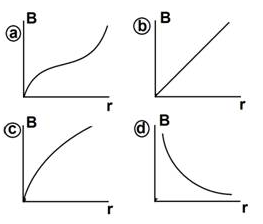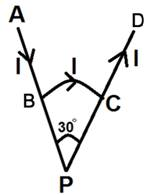# MAGNETIC EFFECTS OF ELECTRIC CURRENT AND MAGNETISM

• Home -
• MAGNETIC EFFECTS OF ELECTRIC CURRENT AND MAGNETISM

MAGNETIC EFFECTS OF ELECTRIC CURRENT AND MAGNETISM - Topic Wise Multiple Choice Questions (MCQ)

Topic Wise free Magnetic Effects of Electric current and magnetism MCQ with solutions for 11th 12th exam Practice Multiple Choice Questions and answer for JEE-mains State CET Engineering Entrance GATE free Online Test

1 )

An element dℓ = dx ↑ (where dx = 1 cm) is placed at the origin and carries a large current I = 10 Amp. What is the mag. field on the Y-axis at a distance of 0.5 meter?

Select correct option from following options

(a) 2 × 10–8 k˄ T            (b) 4 × 108 k˄ T

(c) – 2 × 10–8 k˄ T         (d) – 4 × 10–8 k˄ T

a
b
c
d

View Solution

2 )

Two straight long conductors AOB and COD are perpendicular to each other and carry currents I1 and I2. The magnitude of the mag. field at a point "P" at a distance "a" from the point "O" in a direction perpendicular to the plane ABCD is

(a) [(μ0) / (2πa)](I1 + I2)

(b) [(μ0) / (2πa)](I1 – I2)

(c) [(μ0) / (2 πa)](I12 + I22)1/2

(d) [(μ0) / (2 πa)][(I1I2) / (I1 – I2)]

a
b
c
d

View Solution

3 )

B→R graph. The mag. field B at a distance r from a long straight wire carrying a current varies with r as shown in Fig.a
b
c
d

View Solution

4 )

A current path shaped as shown in figure produces a mag. field at point ''P'', the centre at the arc BC. If the arc subtends an angle of 30° and the radius of the arc is 0.6 meter. What is the magnitude of the field at point P if the current is 3 AMP?Select correct option from the following options

(a) 2.62 × 10–6 T            (b) 2.62 × 10–7 T

(c) 3.62 × 10–7 T            (d) 2.62 × 10–8 T

a
b
c
d

View Solution

5 )

A length L of wire carries a steady current 1. It is bent first to form a coil of 1 turn. The same length is now bent more sharply to give a double loop of smaller radius. The magnetic field at the centre caused by the same current is _________

A quarter of its first value
Unchanged
Four times of its first value
A half of its first

View Solution